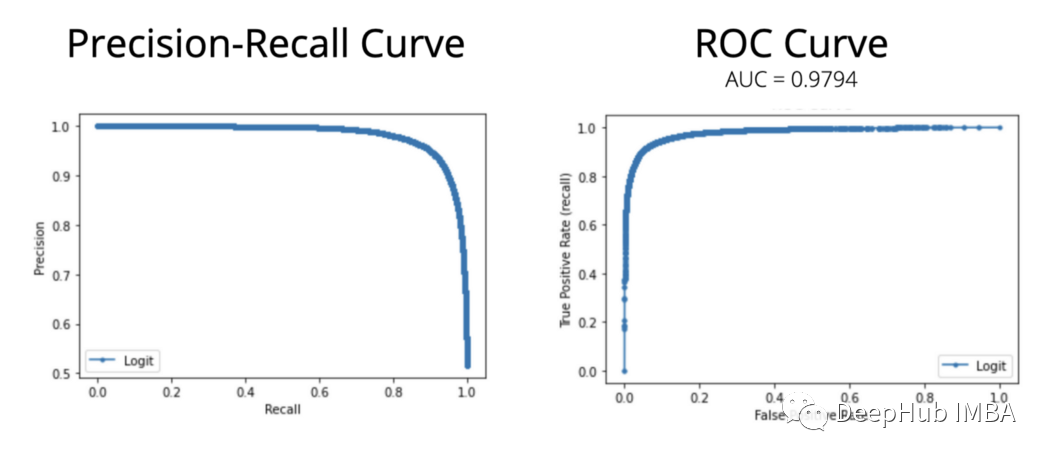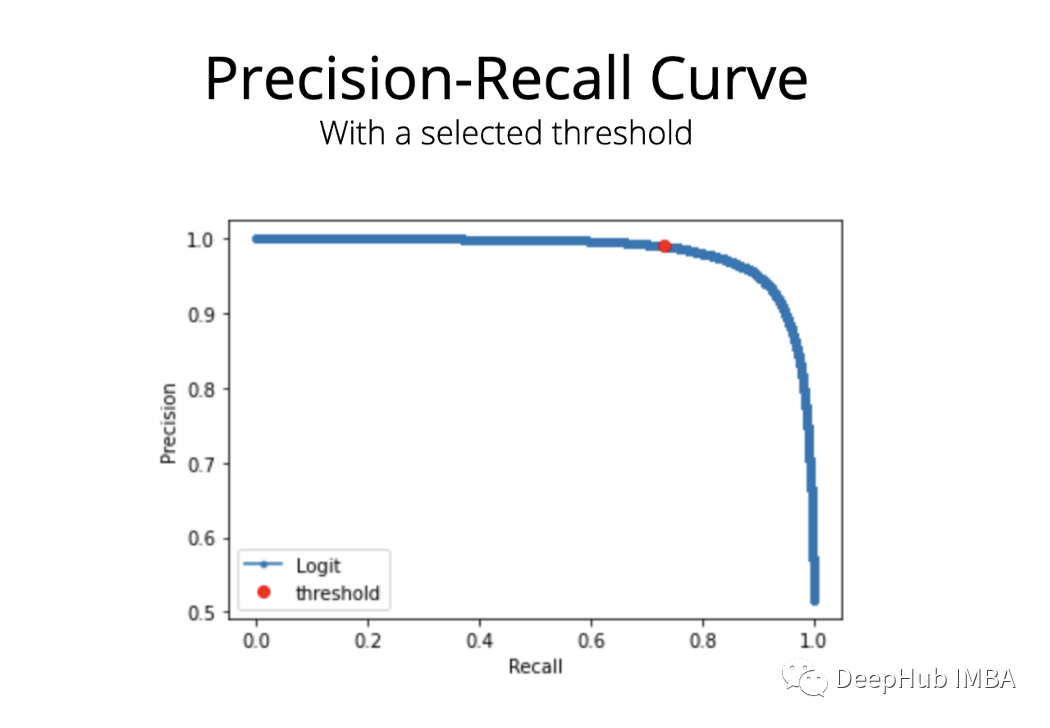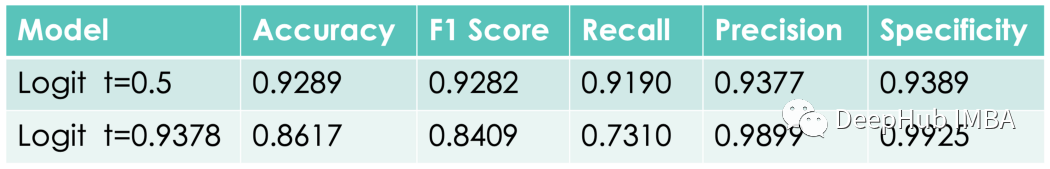0

# 使用阈值调优改进分类模型性能## 用于分类的常用指标

F1 分数F1 score：Precision 和 Recall 之间的调和平均值。

(ROC) 曲线：该曲线显示了真正例率和假正例率之间的权衡。代表模型的性能。

ROC曲线下面积（AUC）：ROC曲线下面积。如果这个面积等于 1，我们就有了一个完美的分类器。如果它等于 0.5，那么就是一个随机的分类器。

Precision-Recall曲线：这条曲线显示了不同阈值下的精度和召回值。它用于可视化 Precision 和 Recall 之间的权衡。

`````` def make_classification_score(y_test, predictions, modelName):
tn, fp, fn, tp = confusion_matrix(y_test, predictions).ravel() # ravel() used to convert to a 1-D array
prec=precision_score(y_test, predictions)
rec=recall_score(y_test, predictions)
f1=f1_score(y_test, predictions)
acc=accuracy_score(y_test, predictions)
# specificity
spec=tn/(tn+fp)

score = {'Model': [modelName], 'Accuracy': [acc], 'f1': [f1], 'Recall': [rec], 'Precision': [prec], \
'Specificity': [spec], 'TP': [tp], 'TN': [tn], 'FP': [fp], 'FN': [fn], 'y_test size': [len(y_test)]}
df_score = pd.DataFrame(data=score)
return df_score
``````

## “预测概率”技巧

`````` def probs_to_prediction(probs, threshold):
pred=[]
for x in probs[:,1]:
if x>threshold:
pred.append(1)
else:
pred.append(0)
return pred
``````

## 阈值优化

• 计算ROC AUC，它等于0.9794
• 计算并绘制ROC曲线
• 计算并绘制精度-召回率曲线

`````` def probs_to_prediction(probs, threshold):
pred=[]
for x in probs[:,1]:
if x>threshold:
pred.append(1)
else:
pred.append(0)
return pred

# getting predicted probability values
probability = model.predict_proba(X_test)

# calculate ROC AUC score. AUC = 0.9794
print("Logit: ROC AUC = %.4f" % roc_auc_score(y_test, probability[:, 1]))

# calculate and plot the ROC curve
model_fpr, model_tpr, _ = roc_curve(y_test, probability[:, 1])
plt.plot(model_fpr, model_tpr, marker='.', label='Logit')
plt.xlabel('False Positive Rate')
plt.ylabel('True Positive Rate (recall)')
plt.legend()
plt.title("ROC Curve")
plt.show()

# calculate and plot the Precision-Recall curve
model_precision, model_recall, thresholds = precision_recall_curve(y_test, probability[:, 1])
plt.plot(model_recall, model_precision, marker='.', label='Logit')
plt.xlabel('Recall')
plt.ylabel('Precision')
plt.legend()
plt.title('Precision-Recall Curve')
plt.show()
```````````` plt.plot(model_recall, model_precision, marker='.', label='Logit')
plt.plot(model_recall, model_precision, "ro", label="threshold")
plt.xlabel('Recall')
plt.ylabel('Precision')
plt.legend()
plt.title('Precision and Recall values for a chosen Threshold')
plt.show()
```````````` print("Threshold value = %.4f" % thresholds)

# results with the chosen threshold
predictions = probs_to_prediction(probability, thresholds)
make_classification_score(y_test, predictions, "logit, custom t")
``````## 总结

2022-09-16T11:15:26+08:00 已修改

### “使用阈值调优改进分类模型性能”的评论:

##### 关于作者##### Deephub